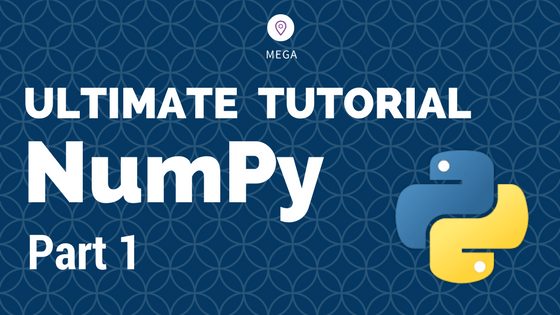## Numpy Tutorial – Your first numpy guide to build python coding foundations

This is part 1 of the numpy tutorial covering all the core aspects of performing data manipulation and analysis with numpy’s ndarrays. Numpy is the most basic and a powerful package for scientific computing and data manipulation in python. Numpy Tutorial Part 1: Introduction to Arrays. Photo by Bryce Canyon. Also Read: Numpy Tutorial – …